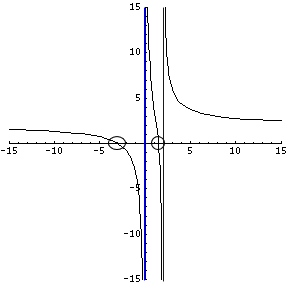# Isn't root a biology term??

### What is a "root"?

A root is a value for which a given function equals zero. When that function is plotted on a graph, the roots are points where the function crosses the x-axis.

For a function, $$f(x)$$, the roots are the values of x for which $$f(x)=0$$. For example, with the function $$f(x)=2-x$$, the only root would be $$x = 2$$, because that value produces $$f(x)=0$$.

Of course, it's easy to find the roots of a trivial problem like that one, but what about something crazy like this:

$$f(x)=\frac{(2x-3)(x+3)}{x(x-2)}$$

### Steps to find roots of rational functions

1. Set each factor in the numerator to equal zero.

2. Solve that factor for x.

3. Check the denominator factors to make sure you aren't dividing by zero!

### Numerator Factors

Remember that a factor is something being multiplied or divided, such as $$(2x-3)$$ in the above example. So, the two factors in the numerator are $$(2x-3)$$ and $$(x+3)$$. If either of those factors can be zero, then the whole function will be zero. It won't matter (well, there is an exception) what the rest of the function says, because you're multiplying by a term that equals zero.

So, the point is, figure out how to make the numerator zero and you've found your roots (also known as zeros, for obvious reasons!). In this example, we have two factors in the numerator, so either one can be zero. Let's set them (separately) equal to zero and then solve for the x values:

$$2x - 3 = 0$$ $$2x = 3$$ $$x = \frac{3}{2}$$

AND

$$x + 3 = 0$$ $$x = -3$$

So, $$x = \frac{3}{2}$$ and $$x = -3$$ become our roots for this function. They're also the x-intercepts when plotted on a graph, because y will equal 0 when x is 3/2 or -3.

### Denominator Factors

Just like with the numerator, there are two factors being multiplied in the denominators. They are $$x$$ and $$x-2$$. Let's set them both equal to zero and solve them:

$$x = 0$$

AND

$$x - 2 = 0$$ $$x = 2$$

Those are not roots of this function. Look what happens when we plug in either 0 or 2 for x. We get a zero in the denominator, which means division by zero. That means the function does not exist at this point. In fact, x = 0 and x = 2 become our vertical asymptotes (zeros of the denominator). So, there is a vertical asymptote at x = 0 and x = 2 for the above function.

Here's a geometric view of what the above function looks like including BOTH x-intercepts and BOTH vertical asymptotes:### Summary

Roots of a function are x-values for which the function equals zero. They are also known as zeros. When given a rational function, make the numerator zero by zeroing out the factors individually. Check that your zeros don't also make the denominator zero, because then you don't have a root but a vertical asymptote.